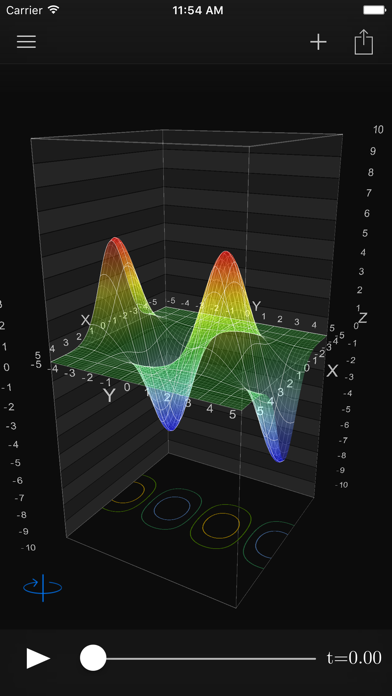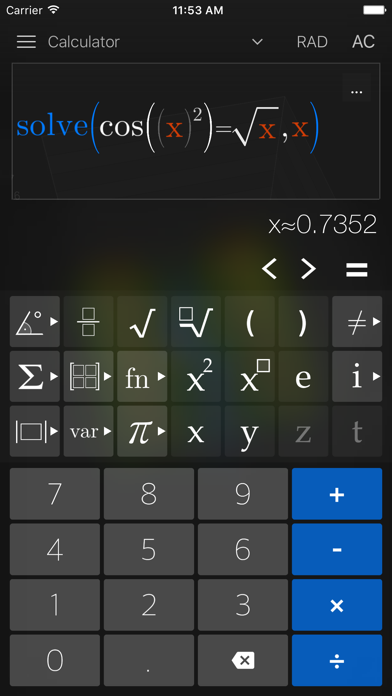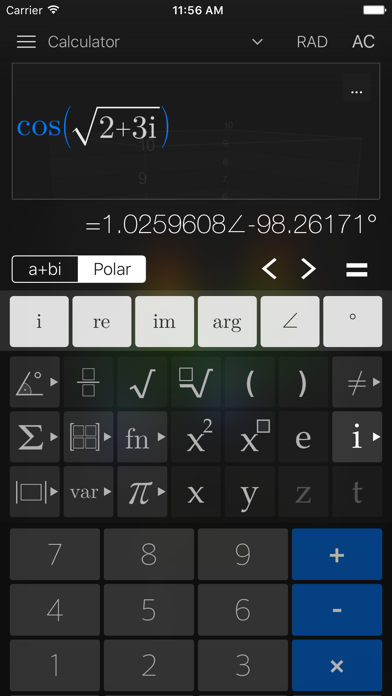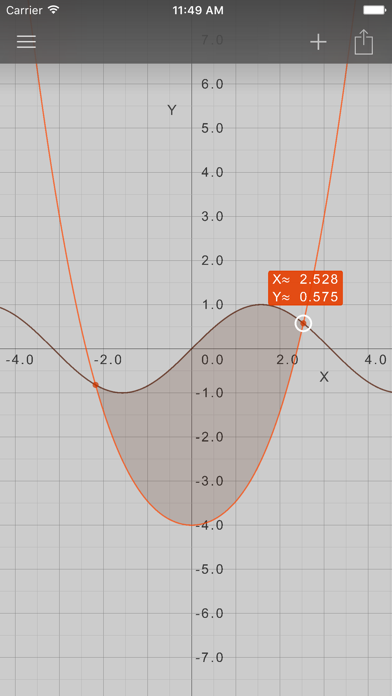## Visual Math 4D - Lumos Educational App Store4.635231
Price -\$1.99
\$1.99

#### DESCRIPTION:

Visual Math 4D is a graphical calculator that allows you to visualize and solve your mathematical equations. It supports various types of equations, such as polar, spherical, parametric and implicit equations, which can be visualized and animated in 2D and 3D. You can also plot and animate vector fields in 2D and 3D. Features: - solve equations - plot cartesian functions with intersections - plot polar- and spherical functions - plot parametric equations in 2D and 3D - plot complex functions (draw real part & imaginary part) - plot vector fields in 2D and 3D - plot implicit equations in 2D an

#### OVERVIEW:

Visual Math 4D is a free educational mobile app By Ronny Weidemann.It helps students practice the following standards .

This page not only allows students and teachers download Visual Math 4D but also find engaging Sample Questions, Videos, Pins, Worksheets, Books related to the following topics.

Developer: Ronny Weidemann

Developer URL: https://www.appnova.de

Software Version: 5.3

Category: Education

Release Date: 2013-06-15T07:56:35Z#### Are you the Developer?EdSearch WebSearch